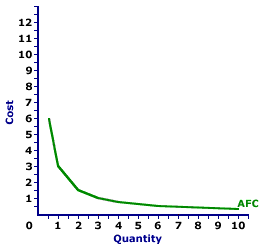Tuesday  March 28, 2023
 AmosWEB means Economics with a Touch of Whimsy!VERTICAL EQUITY: A system of taxes that treats unequal people unequally. In other words, if you make the less income than someone else and pay fewer personal income taxes, then we have vertical equity.AVERAGE FIXED COST CURVE:

A curve that graphically represents the relation between average fixed cost incurred by a firm in the short-run product of a good or service and the quantity produced. This curve is constructed to capture the relation between average fixed cost and the level of output, holding other variables, like technology and resource prices, constant. The average fixed cost curve is one of three average curves. The other two are average total cost curve and average variable cost curve. A related curve is the marginal cost curve.
Average Fixed Cost CurveThe average fixed cost curve is negatively sloped. Average fixed cost is relatively high at small quantities of output, then declines as production increases. The more production increases, the more average fixed cost declines. The reason behind this perpetual decline is that a given FIXED cost is spread over an increasingly larger quantity of output.

The graph to the right is the average fixed cost curve for the short-run production of Wacky Willy Stuffed Amigos (those cute and cuddly armadillos and tarantulas). The quantity of Stuffed Amigos production, measured on the horizontal axis, ranges from 0 to 10 and the average fixed cost incurred in the production of Stuffed Amigos, measured on the vertical axis, ranges from a high of \$6 to a low of \$0.30. Actually, if the quantity is extended beyond 10 Stuffed Amigos, then average fixed cost is less than \$0.30. Or if the quantity is reduced below 1/2 unit, then average fixed cost is greater than \$6. For the geometrically inclined, this average fixed cost curve is a rectangular hyperbola.

This declining average fixed cost curve is a major reason that the average total curve is negatively sloped for relatively small output quantities. In fact, firms that use a lot of fixed inputs relative to variable inputs, such that fixed cost is a substantial share of total cost, spend a lot of their production time in the decreasing portion of the average total cost curve. This has a big impact on how these firms operate. If average total cost declines with additional production, then a firm can profitably charge a lower price with increased output.

 <= AVERAGE FIXED COST AVERAGE-MARGINAL RELATION =>Recommended Citation:

AVERAGE FIXED COST CURVE, AmosWEB Encyclonomic WEB*pedia, http://www.AmosWEB.com, AmosWEB LLC, 2000-2023. [Accessed: March 28, 2023].

Check Out These Related Terms...

Or For A Little Background...

And For Further Study...
Search Again?BROWN PRAGMATOX[What's This?] Today, you are likely to spend a great deal of time wandering around the shopping mall seeking to buy either a remote controlled ceiling fan or a how-to book on home decorating. Be on the lookout for empty parking spaces that appear to be near the entrance to a store.Your Complete ScopeA lump of pure gold the size of a matchbox can be flattened into a sheet the size of a tennis court!"Do not go where the path may lead, go instead where there is no path and leave a trail."-- Ralph Waldo EmersonBOABasic Ordering AgreementA PEDestrian's Guide Xtra CreditTell us what you think about AmosWEB. Like what you see? Have suggestions for improvements? Let us know. Click the User Feedback link.| | | | | | | | | | |
| | | |

Thanks for visiting AmosWEB